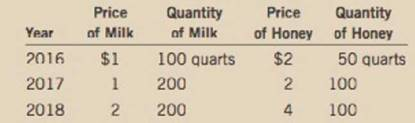Chapter 5, Problem 5PA

Chapter
Section
Textbook Problem

Below are some data from the land of milk and honey.a. Compute nominal GDP, real GDP, and the GDP deflator for each year, using 2016 as the base year.b. Compute the percentage change in nominal GDP, real GDP, and the GDP deflator in 2017 and 2018 from the preceding year. For each year, identify the variable that does not change. Explain why your answer makes sense.c. Did economic well-being increase more in 2017 or 2018? Explain.

Sub part (a):

To determine

The nominal and real GDP and GDP deflator.

Explanation

The GDP is the summation of the monetary value of all the goods and services produced within the political boundary of a country, within a financial year. There are two different ways of calculating the GDP of the economy and they are the real GDP and the nominal GDP. The real GDP is the GDP calculated at the constant prices. There will be a base price index and the value of goods and services will be calculated on the basis of the constant prices. Thus, it will measure the GDP of the economy on the same base year price index, which will help us to identify the inflation in the economy. The nominal GDP is the GDP calculated at the current prices. The GDP will be calculated by multiplying the quantity of goods and services produced with the current year’s market prices which will include the inflation impact.

The nominal GDP of the economy can be calculated by multiplying the quantity produced by the per unit price of the commodity. The quantity produced and price in 2016 was 100 quarts of milk and 50 quarts of honey. The prices were $1 and$2 respectively, for each quart. Thus, the nominal GDP of 2016 can be calculated as follows:

Nominal GDP2016=[(Quantity produced2016×per unit price2016)Milk+(Quantity produced2016×per unit price2016)Honey]=(100×1)+(50×2)=100+100=200

Thus, the nominal GDP of 2016 is $200. Similarly, the quantity produced and price in 2017 was 200 quarts of milk and 100 quarts of honey. The prices were$1 and $2, respectively. Thus, the nominal GDP of 2017 can be calculated as follows: Nominal GDP2017=[(Quantity produced2017×per unit price2017)Milk+(Quantity produced2017×per unit price2017)Honey]=(200×1)+(100×2)=200+200=400 Thus, the nominal GDP of 2017 is$400.

Similarly, the quantity produced in 2018 was 200 quarts of milk and 100 quarts of honey but the prices increased to $2 and$4, respectively. Thus, the nominal GDP of 2018 can be calculated as follows:

Nominal GDP2018=[(Quantity produced2018×per unit price2018)Milk+(Quantity produced2018×per unit price2018)Honey]=(200×2)+(100×4)=400+400=800

Thus, the nominal GDP of 2018 is \$800

Sub part (b):

To determine

The Percentage change in nominal and real GDP and GDP deflator.

Sub part (c):

To determine

Economic well-being.

Still sussing out bartleby?

Check out a sample textbook solution.

See a sample solution

The Solution to Your Study Problems

Bartleby provides explanations to thousands of textbook problems written by our experts, many with advanced degrees!

Get Started

Find more solutions based on key concepts